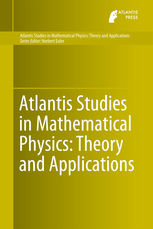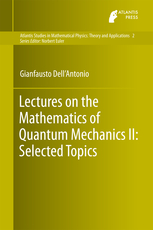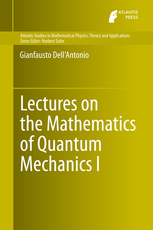# Atlantis Studies in Mathematical Physics: Theory and ApplicationsISSN (Online):
2211-8063
ISSN (Print):
2211-8055
Series Editor(s):

Norbert Euler

The aim of this book series is to publish high-quality monographs in mathematical physics of both theoretical and applied subjects. The main topics are listed below. We expect the books of this series to reflect the current state of the art of some of the popular ongoing research topics in mathematical physics. In addition, we aim to focus on books that make some of the abstract theories and methodologies accessible to applied scientists, such as biologists and engineers, including graduate students in the subjects.

The series is seeking high-quality, original and comprehensive edited or authored text books, lecture notes, conference proceedings, monographs and case studies on topics leveraging all aspects of linear and nonlinear mathematical physics. Topics include:

• methods and applications of nonlinear differential- and difference equations
• classification and applications of integrable systems
• integrability and geometry
• dynamical systems
• many-body problems
• special functions of mathematical physics and q-analysis
• symmetry analysis of differential- and difference equations
• computer algebra and quantum computing with applications
• super-symmetries
• representations and Lie algebras
• nonlinear mathematical biology, including biophysics and mathematical theory of evolution
• problems in applied mathematics, fluid dynamics, engineering sciences and financial mathematics

## Volumes in this SeriesVolume 2

Title: Lectures on the Mathematics of Quantum Mechanics II: Selected Topics
Author(s): Gianfausto Dell'Antonio